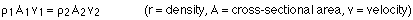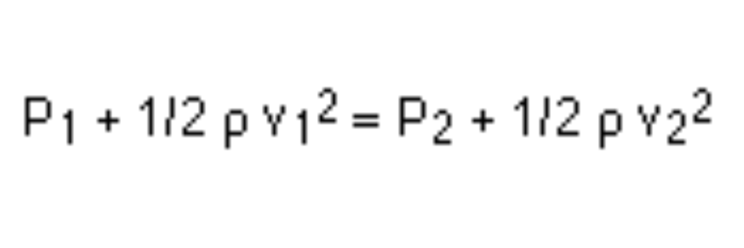# The Complex Physics of How Water Flows Through Pipes

Water pipes are vital to modern society, and they involve a surprising amount of physics and engineering.All around you are pipes that carry water and other liquids to where they need to go. Water and sewage pipes are the unseen heroes that allow modern infrastructure to function easily.

However, you might be surprised that the physics of pipes isn’t as straightforward as you might think. In fact, people go to school to earn entire engineering degrees related to how water flows through pipes (civil engineers). There’s actually some pretty interesting physics going on inside pipes, so let’s take a look and learn a little bit more about how fluid flows through these liquid highways.

## Fluid dynamics and assumptions

Discussing the physics of pipes is really a discussion of fluid dynamics, which is the study of how fluids behave while in motion. Fluid dynamics isn’t the least complicated thing in the world, so we’ll stick to the basics for this article.

Any given fluid can flow in either a steady-state or the fluid can be turbulent. A steady flow implies that the fluid maintains constant velocity at any given point, whereas turbulent flow implies the fluid changes speed and direction all throughout a pipe, or flow. Steady flow is easy to represent, just straight lines for motion and velocity, etc.

Expressing turbulent flow is best done by handing a toddler a marker and telling them to scribble. This is how the experts do it.

RELATED: HOW ENGINEERS CALCULATE THE POLLUTION AND PHYSICS OF SMOKESTACKS

In reality, though, turbulent flow just means the properties of the flow are constantly changing, and this makes it hard to model.

Fluids may be compressible or incompressible. Liquids are generally considered incompressible. A gallon or liter of water can’t easily be compressed down into anything smaller. Whereas a gallon or liter of air could be compressed to much smaller volumes.

Since we’re focusing on water pipes in this article, we’ll just assume all the fluids flowing through the pipes are incompressible.

Fluids also have varying degrees of viscosity. We can think of viscosity a bit like thickness. Viscous fluids flow slowly, and non-viscous fluids flow more easily. Honey flowing through a pipe would look and act a lot different compared to water flowing through a pipe.

Fluid flow can also be rotational or irrotational. An irrotational flow occurs when the flow undergoes a non-net rotatory motion

Now that we’ve defined the terms involved, we’re going to make some assumptions, because like I said, fluid dynamics is complicated.

To make this simpler, we will assume an irrotational, incompressible, steady streamline, non-viscous flow. We are making all of these assumptions because if we don’t, the math get’s squirrely, and fast.

## The math of water pipes

The first equation we’ll need to learn is the continuity equation or the equation of continuity. This equation states that for an incompressible fluid flowing through a tube/pipe of variable cross-section, with one inlet and one outlet, the mass flow rate is the same everywhere in the tube. The mass flow rate is simply the rate at which a given weight of water flows through the tube. It is equal to the total mass of the fluid divided by the time interval.

Expressing that statement in simpler terms, the mass of water flowing through a pipe is assumed to be a constant, regardless of the cross-sectional area.

The equation we get out of that is:The density of the fluid, rho, is generally constant in a fluid in a pipe. A is the cross-sectional area, which depends upon the size of the pipe, and V is the velocity. So using this equation, you could determine how fast a fluid would flow through a pipe of a given size, and also how large you need to make a pipe to get a fluid to flow at a given speed.

But let’s back up for a second, how do you even make a fluid flow through a pipe? Well, you’re going to need to use either gravity or a pump. Gravity just means tilting a pipe downward so gravity acts on the fluid, causes it to accelerate, and gets it moving through the pipe. This is how sewer systems work in most cases.

The second way is by creating a pressure differential. This is generally achieved through using pumps. Utilizing pressure differentials, you can apply greater pressure to a fluid at one end of a pipe, which will cause it to flow to the end with the lower pressure.

When it comes to pipes, fluid, and pressure, remember, the fluid wants to have equal pressure everywhere, if it could. When there are a differential and a path of flow, the fluid will go to the lower pressure (under the conditions we assumed).

So how can we model this flow through a pipe? We can use something called Bernoulli’s equation, which relates the pressure, velocity, and height of a fluid in a pipe. Bernoulli’s equation is everywhere in fluid mechanics, and it’s probably the most important equation in this line of physics. It comes into play in airplanes, sports, and of course, in plumbing pipes.

So what is Bernoulli's equation? It states that the pressure, speed, and height of a fluid at two points in a steady-flow, non-viscous, incompressible fluid are related like this:

P is pressure, Rho is density, V is velocity, y (often h is used instead) is height, and g is gravitational acceleration, which is a constant depending upon elevation/location (generally, you can use 9.81 m/s^2 for this).

Utilizing this equation, you can essentially solve for any one of these variables. This is incredibly helpful to engineers in calculating any number of things.

But what’s interesting about this equation? Well, let’s consider fluid flowing through a horizontal pipe. If the pipe is narrower at one spot, the continuity equation implies that the velocity of the fluid is greater at the narrow section. So is the pressure higher or lower in the narrow section, where the velocity is higher?

RELATED: 35 ENGINEERING INVENTIONS THAT CHANGED THE WORLD

You might think that if the velocity is super high, then the pressure must be super high too. If you stick your hand at the end of a hose nozzle, you’re going to feel a lot of force!

However,in this instance, the force you’re feeling isn’t coming from pressure, it’s coming from your hand taking the momentum out of the fluid.

## Simplifying Bernoulli's equation

We can simplify Bernoulli's equation in a flat pipe, since the height doesn’t change, to look like this:A simplified version of Bernoulli's equations when the aforementioned assumptions are considered.

Since the smaller pipe, on the right, has a larger velocity, the pressure on the right has to be lower in order to get the equation to balance. It’s this difference in pressure that actually causes the fluid to flow faster!

So, that just about wraps up some of the most basic physics and equations surrounding how water or fluids flow through pipes. And these equations can be used in other fluid problems, too. But remember, we made a ton of assumptions here. In the real world, we have to do a whole lot more calculus and complex mathematics to actually calculate things.